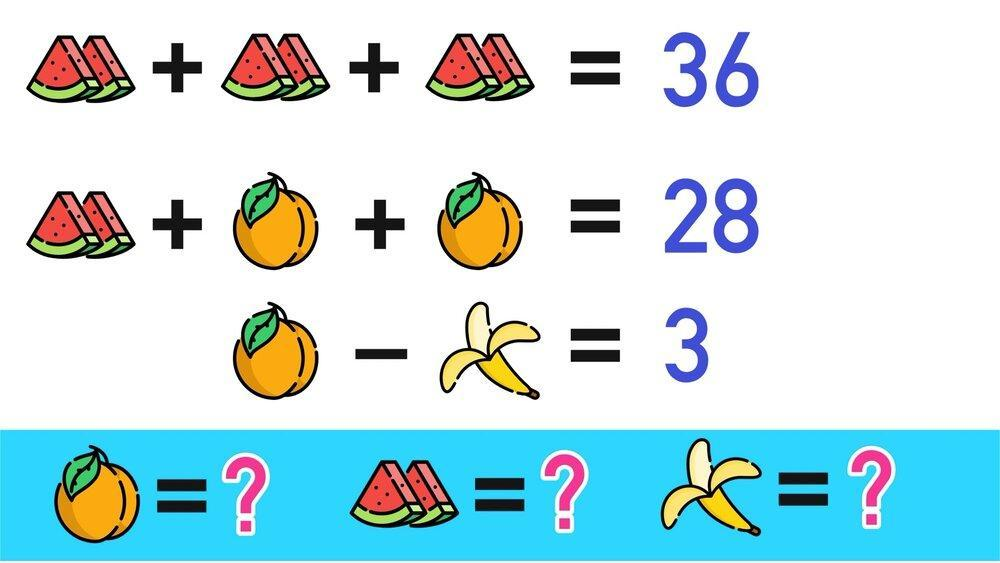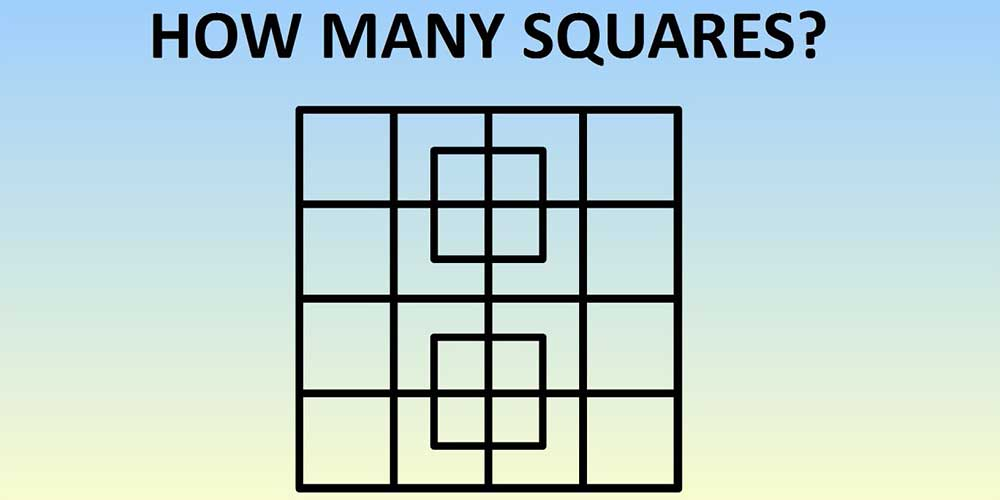# Can You Solve these 3 Impossible Maths Questions?

•## Chapters

There are some questions in maths that everyone gets wrong. Keep on reading to find out why and to test your knowledge.

Some of these questions may look easy but we'll shed some information on why they’re not as easy to solve. 👍## Question 1 – 9 + 3 x 6 ÷ 2 = ?

When you look at this equation above, there will be some who will answer 36, while others will answer 18. But, what is the right answer? Answers to this question often differ completely! 🤔

It all depends on what you learnt was the meaning of the acronym BODMAS -Brackets, orders, division, multiplication, addition, and subtraction. 😅

The order must mean the order of the equation, right? Actually, that's not the case. 😮 Orders refer to the power, square roots, or indices, not to the order of the equation. This is where a lot of people get confused

The answer is actually 18 and not 36. Since the sum should technically read as follows:

9 + (3 x (6 ÷ 2)) =

9 +(3 x 3) =

9 + 9 = 18

##Can you figure out this problem? It’s a challenge! Many can’t do it without using a piece of paper. 💭

If you are studying for A-levels, you’ve probably come across financial problems given as word problems and had to visualise the scenario. This is somewhat similar.

The other problem some children have, is the idea of working backwards, instead of being given the numbers.

With the watermelon 🍉 you have to think backwards. You need to divide 36 by 3 (represents the three watermelons in the picture), instead of being given 12 in the equation. 🤔

Then you have to take 28 from the second equation and subtract 12 from it. Then you divide the remaining number by 2 (since there are 2 peaches). This gives you 16 divided by 2, or 8, which is the value of the peaches. 🍑

Take 8 and subtract 3, giving you 5, which is the value of the banana. 🍌

It all ends up being a mixture of mathematics and visualisation. Alongside working backwords using the power of deduction!

## Question 3 – How many squares can you count?Here is a fun geometry question! Starting with the answer… There are 40 individual squares 🟦 to count in this photo.

Most of you will get this wrong because of one of two reasons. Either you gave up halfway, or you genuinely did not count a certain part as its own square. 🤷

Start on the outside, meaning one square. Then count all the smallest squares that are within that big square, including those overlapping ones.

There are 18 little squares in total. Those 18 little squares make 9 bigger squares in different places, and then 4 even bigger ones after that. Then in those overlapping squares, you find 8 tiny squares. 🧮

So, let’s break this up for anyone who is lost:

• 1 giant square
• 18 small squares
• 9 medium squares
• 4 big squares
• 8 tiny squares

It comes to 40 squares in total. You can find more similar shapes online and challenge your friend to see who counts the correct number. Use different colours to indicate them on a diagram and whoever finds the most wins! 🤪

## Why are these questions so difficult?

We know it’s easy to blame yourself for giving up on some of the questions, but sometimes it really may be a lack of concrete knowledge taught in school.

It can be scary to see how quickly you forget or how you were taught incorrectly. 😔 This is why it's a great idea to consider hiring a maths tutor.

Book a free maths trial lesson with GoStudent today and free yourself from any maths struggles in the future! 😄